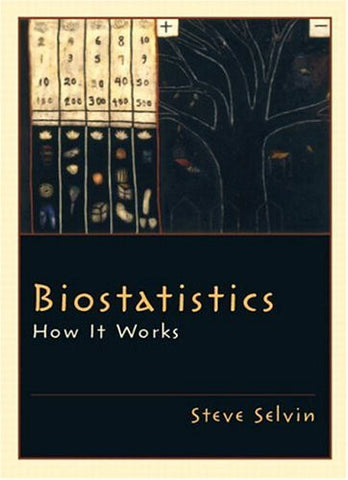Biostatistics: How It Works

• \$64.05
• Save \$132
• Binding: Hardcover
• Author: Steve Selvin
• Publish Date: 2003-07-19

Attention : For textbook, access codes and supplements are not guaranteed with used items.

A clear, consistent, and rigorous approach to statistics at an elementary level, this book's goal is to provide a sophisticated introduction of how and why the statistics process works. Every concept is carefully and clearly explained, enriched by a mathematical/statistical justification, and then illustrated by at least one concrete, worked example. Beginning with basic concepts, the book allows readers to acquire the ability to understand rather complicated statistical issues, such as linear regression theory and application. After introducing the sample mean and a few other descriptive statistics, the book turns to elementary probability theory, then extends to characterize samples selected from populations. It then explores the accuracy and precision of the mean value calculated from samples, and then presents the chi-square analytic technique. The remainder of the book deals with summarizing and analyzing bivariate data. For beginners interested in statistical methods to understand data analysis; these readers are employed in many areas, including Public Health, Genetics, Forestry, Molecular Biology, Epidemiology, Biophysics, medical researchers, and pre-med students.